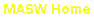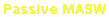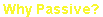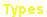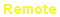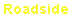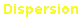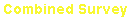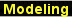e-mail: contact@masw.com
Modeling Scheme for Passive Surface Waves (from Park and Miller, 2008)

The following scheme generates a synthetic seismogram of surface waves from given dispersion curve(s) and source-receiver location information.  It is not a modeling
scheme starting from a layered earth model.  Its main utility is to examine a dispersion analysis scheme to process passive surface waves in association with such source
characteristics as location, spectral content, relative power between different modes, repetition, etc., and also with receiver array characteristics such as shape, size, number
of channels used, etc.  It calculates arrival times of all the frequencies being considered at each receiver location.  Geometric (cylindrical) damping and attenuation (Q-factor)
effects are taken into account as an amplitude-modulation factor.  In the case of multiple sources and modes, the same calculation is repeated independently for each source
and each mode, and then individual results are vertically stacked together to constitute the final seismogram (seismic trace).  Mathematical formulations are described below
and a sample modeling is illustrated in
Fig. 1.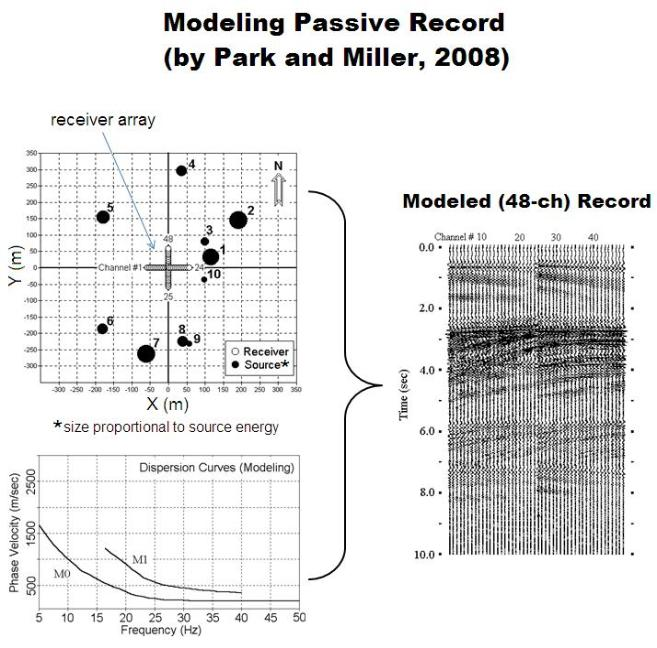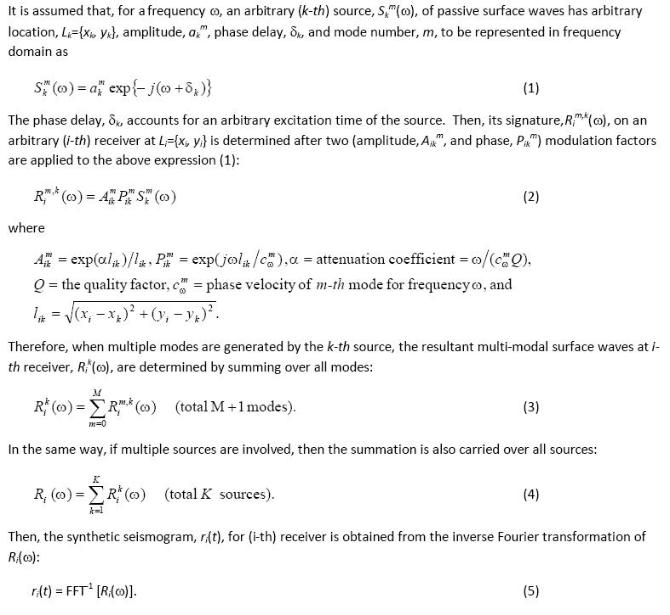Fig. 1.  A sample modeling with a 48-channel cross receiver array and ten
different source points of different intensities scattered around the array.  Two
dispersion modes are incorporated in the modeling to generate the 10-sec long
field record.